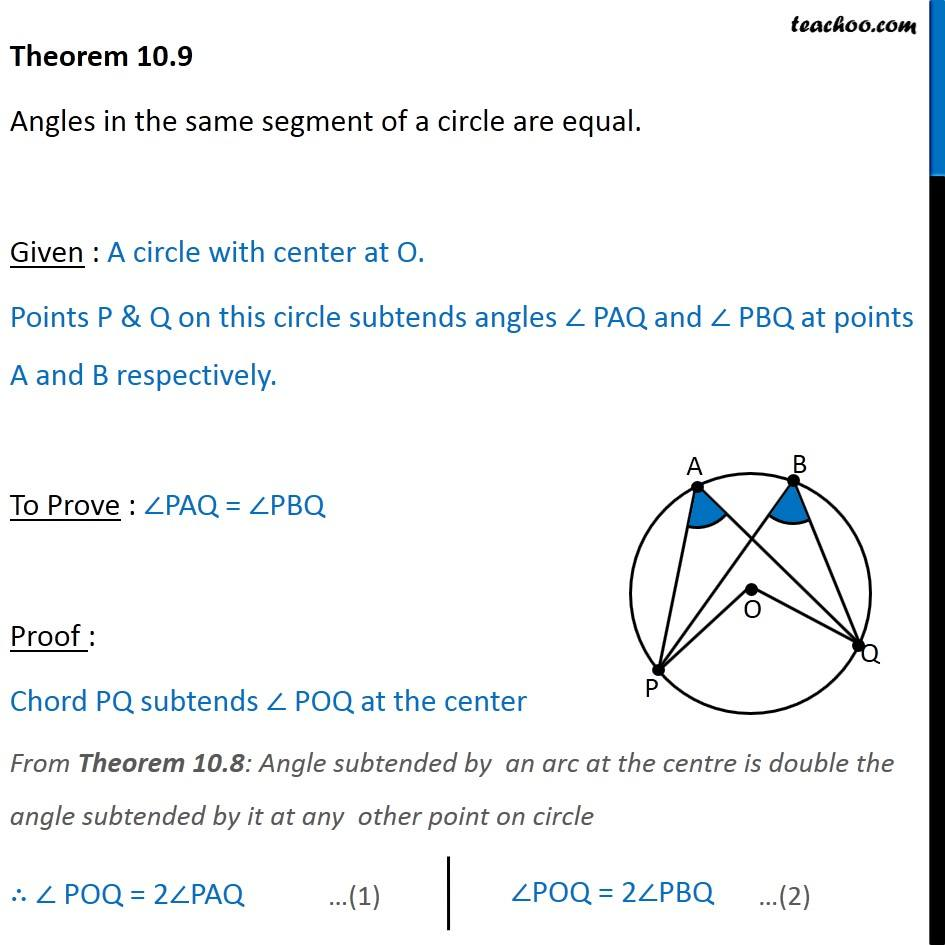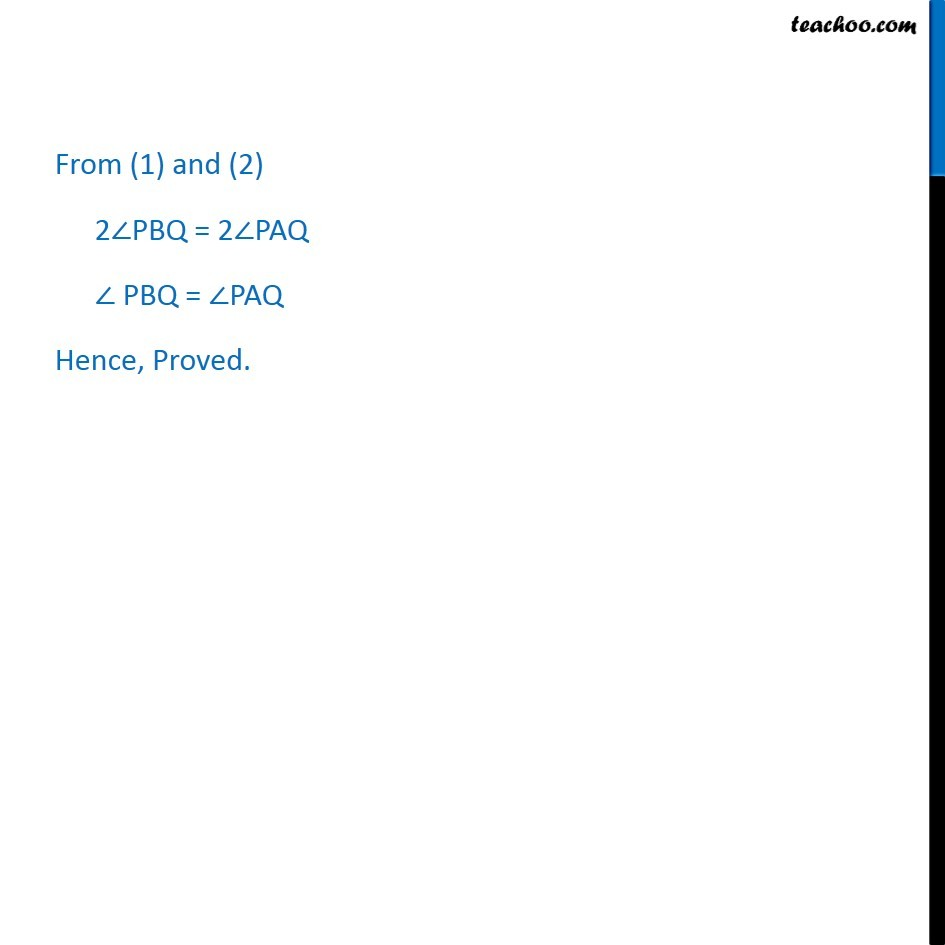Theorems

Chapter 10 Class 9 Circles (Term 2)
Serial order wise### Transcript

Theorem 10.9 Angles in the same segment of a circle are equal. Given : A circle with center at O. Points P & Q on this circle subtends angles ∠ PAQ and ∠ PBQ at points A and B respectively. To Prove : ∠PAQ = ∠PBQ Proof : Chord PQ subtends ∠ POQ at the center From Theorem 10.8: Angle subtended by an arc at the centre is double the angle subtended by it at any other point on circle ∴ ∠ POQ = 2∠PAQ ∠POQ = 2∠PBQ From (1) and (2) 2∠PBQ = 2∠PAQ ∠ PBQ = ∠PAQ Hence, Proved.